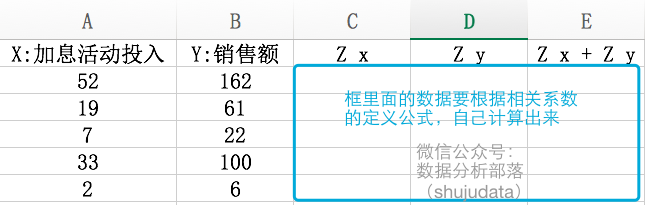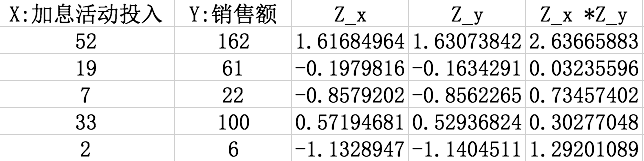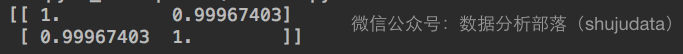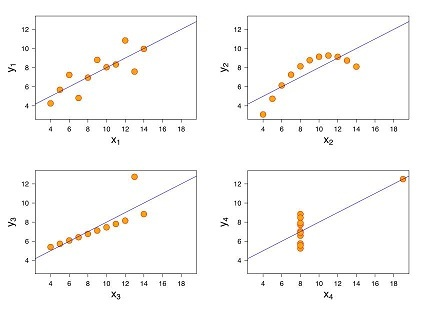基础铺垫

• 相关系数（correlation coefficient）

• 相关系数是变量间关联程度的最基本测度之一，如果我们想知道两个变量之间的相关性，那么我们就可以计算相关系数，进行判定。
• 相关系数基本特征

• 方向

• 正相关：两个变量变化方向相同

• 负相关：两个变量变化方向相反

• 量级（magnitude）

• 低度相关：0 ≤ ｜r｜< 0.3

• 中度相关：0.3 ≤ ｜r｜< 0.8

• 高度相关：0.8 ≤ ｜r｜< 1

• 散点图

• 在进行相关分析之前，通常会绘制散点图来观察变量之间的相关性，如果这些数据在二维坐标轴中构成的数据点分布在一条直线上的周围，那么就说明变量间存在线性相关关系，如下图所示：

r=(ZXZY)N

• r

• ZXXz

• ZYYz

• NXY

金融场景案例实操1.手工写代码计算相关系数

• 根据相关系数的计算公式，我们首先要计算出每个向量的z分数

• z分数的计算公式：每个值减去向量的均值再除以标准差 ZX=(X-XMean)/XSD
#---author:朱元禄---
import numpy
X = [52,19,7,33,2]
Y = [162,61,22,100,6]

#均值
XMean = numpy.mean(X)
YMean = numpy.mean(Y)

#标准差
XSD = numpy.std(X)
YSD = numpy.std(Y)

#z分数
ZX = (X-XMean)/XSD
ZY = (Y-YMean)/YSD• 根据上面相关系数的计算公司，我们套公式，手工计算一下

r=2.64+0.03+0.73+0.3+1.295=0.9997

• 也可以在python内直接计算：
#相关系数
r = numpy.sum(ZX*ZY)/(len(X))

2.使用numpy的corrcoef方法计算

numpy.corrcoef(X,Y)• 我们可以看到用corrcoef计算的值和我们手工计算的值是一样的，这里计算得到的是一个对称矩阵，对角线的位置都是1，代表向量和本身完全自相关，1行2列和2行1列的值一样，因为第一个向量和第二个向量的相关系数等于第二个向量和第一个向量的相关系数，所以为对称矩阵

3.使用pandas.DataFrame的corr方法计算

import pandas
data = pandas.DataFrame({
'X':X,
'Y':Y
})
data.corr()

更深入的探讨：数据分析的陷阱－安斯库姆四重奏

1.Anscombe’s quartet

• 统计学里大名鼎鼎的Anscombe’s quartet是什么？（jacky有时真搞不懂统计学一些术语的翻译，quartet怎就翻译成了“四重奏”，既然得到了统计大佬们的公认，我也不好多说了）
• 先看下下面四个散点图，这四幅图表述的数据特征差异有对大，在散点图赏是不是一目了然？• 下面我们来计算下它们的统计特性，竟然惊人的一样

X的平均数 9 （数据分析部落）
X的方差 11 （公众号：shujudata）
Y的平均数 7.5
Y的方差 4.12
X与Y之间的相关系数 0.816